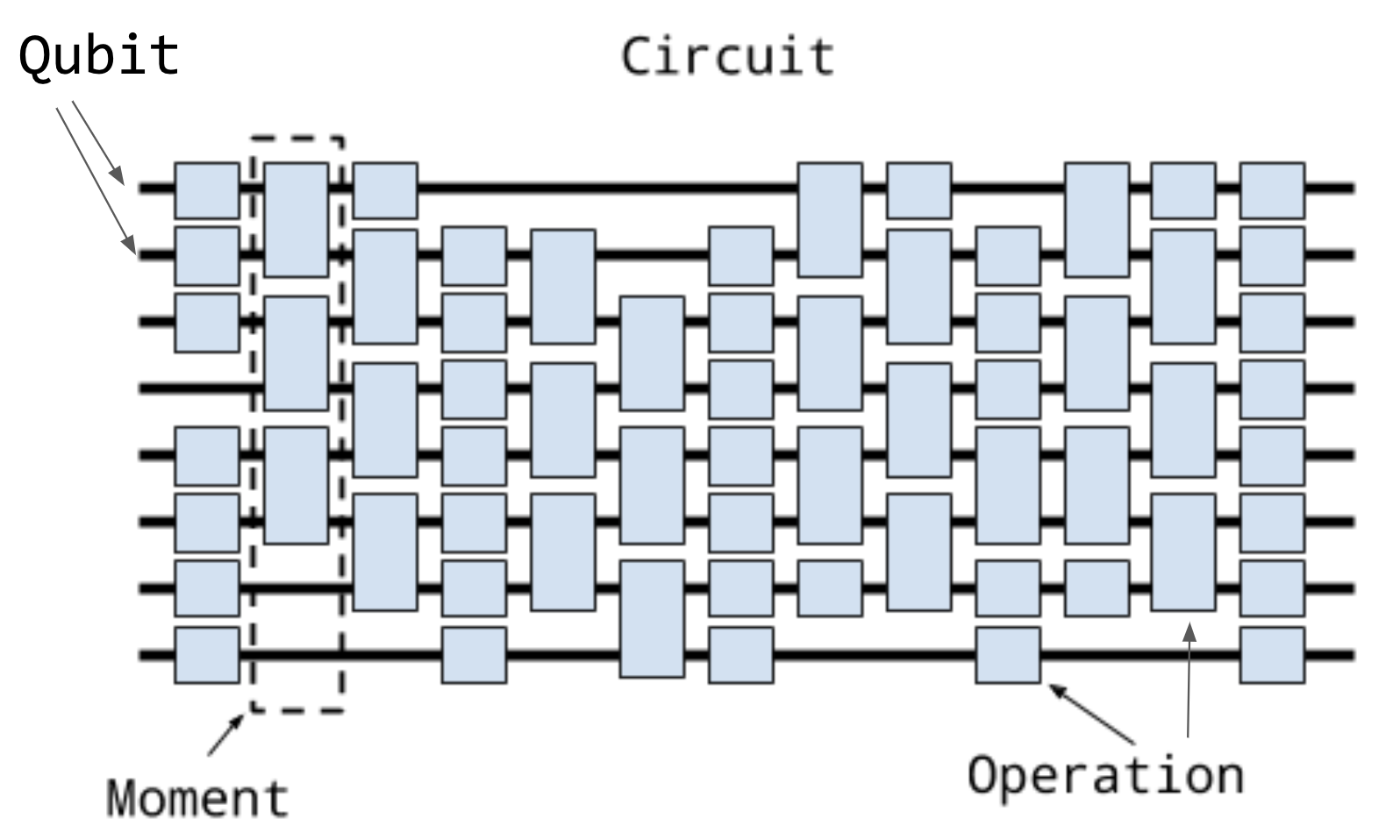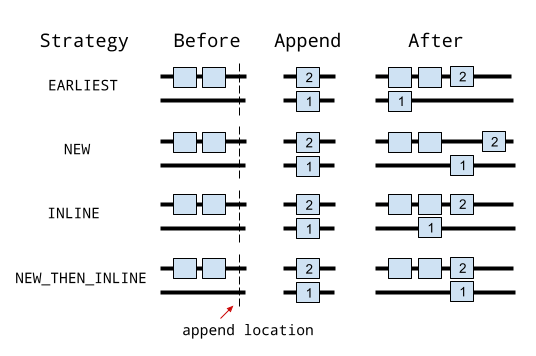Circuits

try:
import cirq
except ImportError:
print("installing cirq...")
!pip install --quiet cirq
print("installed cirq.")

Conceptual overview

The primary representation of quantum programs in Cirq is the Circuit class. A Circuit is a collection of Moments. A Moment is a collection of Operations that all act during the same abstract time slice. An Operation is a some effect that operates on a specific subset of Qubits, the most common type of Operation is a GateOperation.Let's unpack this.

At the base of this construction is the notion of a qubit. In Cirq, qubits and other quantum objects are identified by instances of subclasses of the Qid base class. Different subclasses of Qid can be used for different purposes. For example, the qubits that Google’s Xmon devices use are often arranged on the vertices of a square grid. For this, the class GridQubit subclasses Qid. For example, we can create a 3 by 3 grid of qubits using

import cirq
qubits = [cirq.GridQubit(x, y) for x in range(3) for y in range(3)]

print(qubits)
(0, 0)

The next level up is the notion of a Gate. A Gate represents a physical process that occurs on a Qubit. The important property of a Gate is that it can be applied to one or more qubits. This can be done via the Gate.on method itself or via (), and doing this turns the Gate into a GateOperation.

# This is an Pauli X gate. It is an object instance.
x_gate = cirq.X
# Applying it to the qubit at location (0, 0) (defined above)
# turns it into an operation.
x_op = x_gate(qubits)

print(x_op)
X((0, 0))

A Moment is simply a collection of operations, each of which operates on a different set of qubits, and which conceptually represents these operations as occurring during this abstract time slice. The Moment structure itself is not required to be related to the actual scheduling of the operations on a quantum computer, or via a simulator, though it can be. For example, here is a Moment in which Pauli X and a CZ gate operate on three qubits:

cz = cirq.CZ(qubits, qubits)
x = cirq.X(qubits)
moment = cirq.Moment([x, cz])

print(moment)
X((0, 2)) and CZ((0, 0), (0, 1))

The above is not the only way one can construct moments, nor even the typical method, but illustrates that a Moment is just a collection of operations on disjoint sets of qubits.

Finally, at the top level a Circuit is an ordered series of Moment objects. The first Moment in this series contains the first Operations that will be applied. Here, for example, is a simple circuit made up of two moments:

cz01 = cirq.CZ(qubits, qubits)
x2 = cirq.X(qubits)
cz12 = cirq.CZ(qubits, qubits)
moment0 = cirq.Moment([cz01, x2])
moment1 = cirq.Moment([cz12])
circuit = cirq.Circuit((moment0, moment1))

print(circuit)
(0, 0): ───@───────
│
(0, 1): ───@───@───
│
(0, 2): ───X───@───

Note that the above is one of the many ways to construct a Circuit, which illustrates the concept that a Circuit is an iterable of Moment objects.

Constructing circuits

Constructing Circuits as a series of Moment objects, with each Moment being hand-crafted, is tedious. Instead, we provide a variety of different ways to create a Circuit.

One of the most useful ways to construct a Circuit is by appending onto the Circuit with the Circuit.append method.

from cirq.ops import CZ, H
q0, q1, q2 = [cirq.GridQubit(i, 0) for i in range(3)]
circuit = cirq.Circuit()
circuit.append([CZ(q0, q1), H(q2)])

print(circuit)
(0, 0): ───@───
│
(1, 0): ───@───

(2, 0): ───H───

This appended a new moment to the qubit, which we can continue to do:

circuit.append([H(q0), CZ(q1, q2)])

print(circuit)
(0, 0): ───@───H───
│
(1, 0): ───@───@───
│
(2, 0): ───H───@───

In these two examples, we appended full moments, what happens when we append all of these at once?

circuit = cirq.Circuit()
circuit.append([CZ(q0, q1), H(q2), H(q0), CZ(q1, q2)])

print(circuit)
(0, 0): ───@───H───
│
(1, 0): ───@───@───
│
(2, 0): ───H───@───

We see that here we have again created two Moment objects. How did Circuit know how to do this? Circuit's Circuit.append method (and its cousin, Circuit.insert) both take an argument called the InsertStrategy. By default, InsertStrategy is InsertStrategy.NEW_THEN_INLINE.

InsertStrategies

InsertStrategy defines how Operations are placed in a Circuit when requested to be inserted at a given location. Here, a location is identified by the index of the Moment (in the Circuit) where the insertion is requested to be placed at (in the case of Circuit.append, this means inserting at the Moment, at an index one greater than the maximum moment index in the Circuit).

There are four such strategies: InsertStrategy.EARLIEST, InsertStrategy.NEW, InsertStrategy.INLINE and InsertStrategy.NEW_THEN_INLINE.

InsertStrategy.EARLIEST, which is the default, is defined as:

Scans backward from the insert location until a moment with operations touching qubits affected by the operation to insert is found. The operation is added to the moment just after that location.

For example, if we first create an Operation in a single moment, and then use InsertStrategy.EARLIEST, Operation can slide back to this first Moment if there is space:

from cirq.circuits import InsertStrategy

circuit = cirq.Circuit()
circuit.append([CZ(q0, q1)])
circuit.append([H(q0), H(q2)], strategy=InsertStrategy.EARLIEST)

print(circuit)
(0, 0): ───@───H───
│
(1, 0): ───@───────

(2, 0): ───H───────

After creating the first moment with a CZ gate, the second append uses the InsertStrategy.EARLIEST strategy. The H on q0 cannot slide back, while the H on q2 can and so ends up in the first Moment.

Contrast this with InsertStrategy.NEW that is defined as:

Every operation that is inserted is created in a new moment.

circuit = cirq.Circuit()
circuit.append([H(q0), H(q1), H(q2)], strategy=InsertStrategy.NEW)

print(circuit)
(0, 0): ───H───────────

(1, 0): ───────H───────

(2, 0): ───────────H───

Here every operator processed by the append ends up in a new moment. InsertStrategy.NEW is most useful when you are inserting a single operation and do not want it to interfere with other Moments.

Another strategy is InsertStrategy.INLINE:

Attempts to add the operation to insert into the moment just before the desired insert location. But, if there’s already an existing operation affecting any of the qubits touched by the operation to insert, a new moment is created instead.

circuit = cirq.Circuit()
circuit.append([CZ(q1, q2)])
circuit.append([CZ(q1, q2)])
circuit.append([H(q0), H(q1), H(q2)], strategy=InsertStrategy.INLINE)

print(circuit)
(0, 0): ───────H───────

(1, 0): ───@───@───H───
│   │
(2, 0): ───@───@───H───

After two initial CZ between the second and third qubit, we try to insert three H Operations. We see that the H on the first qubit is inserted into the previous Moment, but the H on the second and third qubits cannot be inserted into the previous Moment, so a new Moment is created.

Finally, we turn to a useful strategy to start a new moment and then start inserting from that point, InsertStrategy.NEW_THEN_INLINE

Creates a new moment at the desired insert location for the first operation, but then switches to inserting operations according to InsertStrategy.INLINE.

circuit = cirq.Circuit()
circuit.append([H(q0)])
circuit.append([CZ(q1,q2), H(q0)], strategy=InsertStrategy.NEW_THEN_INLINE)

print(circuit)
(0, 0): ───H───H───

(1, 0): ───────@───
│
(2, 0): ───────@───

The first append creates a single moment with an H on the first qubit. Then, the append with the InsertStrategy.NEW_THEN_INLINE strategy begins by inserting the CZ in a new Moment (the InsertStrategy.NEW in InsertStrategy.NEW_THEN_INLINE). Subsequent appending is done InsertStrategy.INLINE, so the next H on the first qubit is appending in the just created Moment.

Here is a picture showing simple examples of appending 1 and then 2 using the different strategiesPatterns for arguments to append and insert

In the above examples, we used a series of Circuit.appendcalls with a list of different Operations added to the circuit. However, the argument where we have supplied a list can also take more than just list values. For instance:

def my_layer():
yield CZ(q0, q1)
yield [H(q) for q in (q0, q1, q2)]
yield [CZ(q1, q2)]
yield [H(q0), [CZ(q1, q2)]]

circuit = cirq.Circuit()
circuit.append(my_layer())

for x in my_layer():
print(x)
CZ((0, 0), (1, 0))
[cirq.H.on(cirq.GridQubit(0, 0)), cirq.H.on(cirq.GridQubit(1, 0)), cirq.H.on(cirq.GridQubit(2, 0))]
[cirq.CZ.on(cirq.GridQubit(1, 0), cirq.GridQubit(2, 0))]
[cirq.H.on(cirq.GridQubit(0, 0)), [cirq.CZ.on(cirq.GridQubit(1, 0), cirq.GridQubit(2, 0))]]
print(circuit)
(0, 0): ───@───H───H───────
│
(1, 0): ───@───H───@───@───
│   │
(2, 0): ───H───────@───@───

Recall that Python functions with a yield are generators. Generators are functions that act as iterators. In the above example, we see that we can iterate over my_layer(). In this case, each of the yield returns produces what was yielded, and here these are:

• Operations,
• lists of Operations,
• or lists of Operations mixed with lists of Operations.

When we pass an iterator to the append method, Circuit is able to flatten all of these and pass them as one giant list to Circuit.append (this also works for Circuit.insert).

The above idea uses the concept of OP_TREE. An OP_TREE is not a class, but a contract. The basic idea is that, if the input can be iteratively flattened into a list of operations, then the input is an OP_TREE.

A very nice pattern emerges from this structure: define generators for sub-circuits, which can vary by size or Operation parameters.

Another useful method to construct a Circuit fully formed from an OP_TREE is to pass the OP_TREE into Circuit when initializing it:

circuit = cirq.Circuit(H(q0), H(q1))
print(circuit)
(0, 0): ───H───

(1, 0): ───H───

Slicing and iterating over circuits

Circuits can be iterated over and sliced. When they are iterated, each item in the iteration is a moment:

circuit = cirq.Circuit(H(q0), CZ(q0, q1))
for moment in circuit:
print(moment)
H((0, 0))
CZ((0, 0), (1, 0))

Slicing a Circuit, on the other hand, produces a new Circuit with only the moments corresponding to the slice:

circuit = cirq.Circuit(H(q0), CZ(q0, q1), H(q1), CZ(q0, q1))
print(circuit[1:3])
(0, 0): ───@───────
│
(1, 0): ───@───H───

Especially useful is dropping the last moment (which are often just measurements): circuit[:-1], or reversing a circuit: circuit[::-1].

[{ "type": "thumb-down", "id": "missingTheInformationINeed", "label":"Missing the information I need" },{ "type": "thumb-down", "id": "tooComplicatedTooManySteps", "label":"Too complicated / too many steps" },{ "type": "thumb-down", "id": "outOfDate", "label":"Out of date" },{ "type": "thumb-down", "id": "samplesCodeIssue", "label":"Samples / code issue" },{ "type": "thumb-down", "id": "otherDown", "label":"Other" }]
[{ "type": "thumb-up", "id": "easyToUnderstand", "label":"Easy to understand" },{ "type": "thumb-up", "id": "solvedMyProblem", "label":"Solved my problem" },{ "type": "thumb-up", "id": "otherUp", "label":"Other" }]